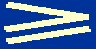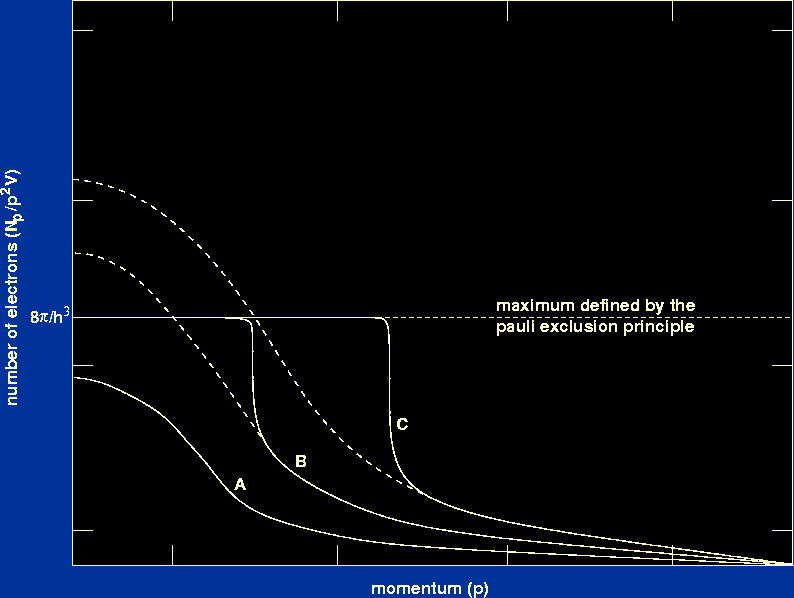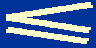Thus far, we have assumed that stars are composed of ideal gases and hence the pressure is given by the ideal gas equation of state we have just derived. We might expect this assumption to break down at sufficiently high densities, when the gas particles in a star are packed so closely together that one can no longer neglect the interactions between them.

One might have thought that electrostatic effects are responsible for the first departures from an ideal gas, but this is not so. In fact, the first such departures are due to interactions between the free electrons in the highly ionised gas because electrons have to obey Pauli's exclusion principle. One way of stating Pauli's exclusion principle is that:

no more than two electrons (of opposite spin) can occupy the same quantum state.

The quantum state of an electron is given by the 6 values which describe its position and momentum, i.e. x, y, z, px, py, pz. It is not possible, however, to know both the position and momentum of an electron with complete accuracy. There is an uncertaintyx in any position coordinate andp in the corresponding momentum coordinate, such thatxph / 4.

This is known as Heisenberg's uncertainty principle and it means that, instead of thinking of a quantum state as a point in six-dimensional position-momentum space (also known as phase space), we can think of a quantum state as a volume, h3, of phase space. Pauli's exclusion principle then tells us that no more than 2 electrons are permitted to occupy each volume h3 of phase space.

Let us now consider what happens at the centre of a star as the density of electrons is increased. The electrons will become increasingly crowded together in position space and eventually a stage will be reached when two electrons with almost the same momenta will occupy almost the same point in position space. This volume of phase space will then be full (according to Pauli's exclusion principle). It will not be possible to squeeze in an additional electron to this region of position space unless its momentum is significantly different (so that it occupies a different region of phase space). The additional electron will therefore possess a higher momentum than it would have had at the same temperature in an ideal gas. Higher momentum implies that such a gas will exert a greater pressure than predicted by the ideal gas equation of state.

 Figure 11: The effect of the Pauli exclusion principle on the Maxwellian distribution of electron momenta.The way in which the Maxwellian distribution of electron momenta is modified by the Pauli exclusion principle is shown in figure 11. Curve A shows the Maxwellian distribution of electron momenta in an ideal gas. At sufficiently high densities, the Maxwellian distribution begins to violate Pauli's exclusion principle (denoted by the horizontal dashed line) at low momenta. These electrons must then occupy higher momentum states than predicted by a Maxwellian distribution. This situation is shown by curve B, where dashed portions of the distribution above the value defined by the Pauli exclusion principle are effectively transferred to higher momenta, as indicated by the solid curve. As the density increases still further, more and more of the low momenta electrons are transferred to higher momentum states, as shown by curve C.

A gas in which the Pauli exclusion principle is important is known as a degenerate gas. Because the ions in such a gas have higher momenta than the electrons, they are less likely to violate Pauli's exclusion principle. The pressure due to the ions can then be treated as an ideal gas, but the pressure due to the degenerate electrons is much larger and hence the gas obeys a different equation of state, which we shall now derive.

Consider a group of electrons occupying a volume V of position space which have momenta lying in the range p and p +p. The volume of momentum space occupied by these electrons is given by the volume of a spherical shell of radius p and thicknessp:

4p2p.

The volume of phase space occupied by these electrons is given by the volume they occupy in position space multiplied by the volume they occupy in momentum space, i.e.

4p2Vp.

The number of quantum states in this volume of phase space is then simply the above volume divided by the volume of a quantum state, h3:

(4p2V / h3)p.

If we now define Npp as the number of electrons in V with momenta in the range p and p +p (so Np is the number of electrons per unit momentum range), Pauli's exclusion principle tells us that:

Npp(8p2V / h3)p.

We will now define a completely degenerate gas as one in which all of the momentum states up to some critical value p0 are filled, while the states with momenta greater than p0 are empty. Strictly speaking, this occurs at absolute zero. Hence, for:

 pp0, Np = 8p2V / h3,   and for p > p0, Np = 0.

The total number of electrons, N, in volume, V, is then given by the integral of Npp, i.e.:

N   =   (8V / h3)p2 dp   =   8p03V / 3 h3.

The pressure, P, of a gas is the mean rate of transport of momentum across unit area. This can be written as follows (see Appendix 3 of Tayler for a derivation):

P = (1/3)(Np / V ) pvp dp,

where vp is the velocity of an electron with momentum p. To evaluate this integral, we must use the relation between p and vp given by the special theory of relativity,

p = mevp / (1 - vp2 / c2) 1/2,

which can be rearranged to give,

vp = (p / me) (1 + p2 / me2c2) -1/2,

where me is the rest mass of an electron. Hence, combining the three expressions for Np, P and vp derived above, we obtain an expression for the pressure of a completely degenerate gas:

P   =   (8/ 3 h3 me)p4 dp / (1 + p2 / me2c2)1/2.

We will not evaluate this integral here (for the solution, see Appendix 3 of Tayler). Instead we will consider two limiting cases:
• a non-relativistically degenerate gas (i.e. p0 << mec)

In this case,

(1 + p2 / me2c2)1/2   ->   1,

and hence

P   =   (8/ 3 h3 me)p4 dp   =   8p05/ 15 h3 me.

Recalling that N = 8p03V / 3 h3 and defining the electron density, ne = N / V, we obtain:

p0 = (h / 2) (3 ne /)1/3.

Combining the last two equations gives:

P   =   (1 / 20) (3 /)2/3 (h2 / me) ne5/3.

• a relativistically degenerate gas (i.e. p0 >> mec). This occurs when the velocity of an electron approaches that of light, in which case its momentum approaches infinity.

In this case, we can neglect 1 in comparison with the p2 / me2c2 term in the pressure equation, i.e.

(1 + p2 / me2c2)1/2   ->   p / mec.

Hence:

P   =   (8c / 3 h3)p3 dp   =   8c p04/ 12 h3.

Recalling that p0 = (h / 2) (3 ne /)1/3 and substituting, we obtain:

P   =   (1 / 8) (3 /)1/3 h c ne4/3.

Our aim is to obtain an equation of state for a degenerate gas in terms of density and chemical composition so that it is compatible with the other equations of stellar structure. Hence we must convert the electron density ne to mass density. We can do this using similar arguments to those given in the derivation of mean molecular weight. For each mass of hydrogen, mH, there is one electron. For helium and heavier elements there is (approximately) half an electron for each mH. Thus:

ne = (X / mH) + ((1 - X) / 2mH) = ((1 + X) / 2mH).

where X is the mass fraction of hydrogen. We can now use this expression to finally write:

 Pgas = K15/3 equation of state of a non-relativistically degenerate gas Pgas = K24/3 equation of state of a relativistically degenerate gas

where

K1 = (h2 / 20 me) (3 /)2/3 [(1 + X) / 2mH]5/3,   and

K2 = (h c / 8) (3 /)1/3 [(1 + X) / 2mH]4/3.

Hence in a degenerate gas, the pressure depends only on the density and chemical composition and is independent of temperature.

Of course, there is not a sharp transition between relativistically degenerate and non-relativistically degenerate gases. Similarly, there is not a sharp transition between an ideal gas and a completely degenerate gas. There is a region of temperature and density in which some intermediate and much more complicated equation of state must be used, a situation known as partial degeneracy.

What types of stars are the above equations applicable to? We will see later in the course that there are stars in which no nuclear fusion is occurring and in which it is the outward-acting force due to degeneracy pressure that balances the inward-acting gravitational force. White dwarfs, brown dwarfs and neutron stars are examples of such stars, in which the degeneracy pressure due to electrons (in the case of white dwarfs and brown dwarfs) or neutrons (in the case of neutron stars) balances the force of gravity. We will also see that many stars temporarily develop degenerate cores as they evolve off the main-sequence.